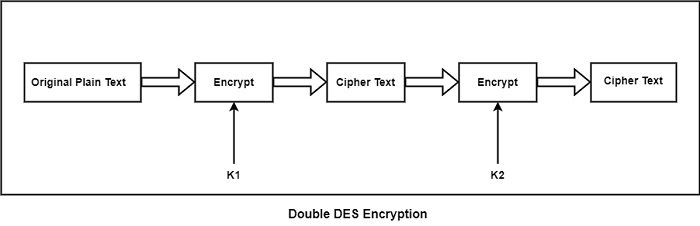# What are the Variations of DES?

Information SecuritySafe & SecurityData Structure

There are two main variations of Data Encryption Standard are as follows −

• Double DES − Double DES is an encryption approach which need two instance of DES on same plain text. In both instances it uses multiple keys to encrypt the plain text. Both keys are needed at the time of decryption.

The 64 bit plain text goes into first DES instance which than transformed into a 64 bit middle text utilizing the first key and thus it goes to second DES instance which provides 64 bit cipher text by utilizing second key.

Double DES is easy as it does that normal DES does. Double DES need two keys to say K1 and K2 in this algorithm. It can implement DES on the original plain text using K1 to receive the encrypted text in cryptography. Therefore, it again implements DES on the encrypted text but this time with the other key K2 in this algorithm.

The final output is the encryption of encrypted text with the original plain text encrypted twice with two different keys as display in the figure −While the doubly encrypted ciphertext block is first decrypted utilizing the key K2 to create singly encrypted ciphertext by plaintext or original text. Therefore, this ciphertext block is decrypted using the key K1 to acquire the original plain text block in cryptography.

Generally, the cryptanalysis for the basic version of DES needed a search of 256 thus the assumption is Double DES needed 2128 keys which is not true for the message. Therefore, a Meet-in-the-middle attack is the disadvantage of double DES in this. Usually, this attack contains encryption from one end, decryption from the other and connecting the results in the middle therefore the name in the message.

• Triple DES − There are two versions of Triple DES which are as follows −

Triple DES with Two Keys − While in triple DES with two keys there are only two keys K1 used by the first and third process and K2 used in the second process in this.

Basically, first, the plain text is encrypted with key K1 then the output of step one is decrypted with K2 and final the output second step is encrypted with key K1 in cryptography. It is also known as encrypt decrypt encrypt (ECE) mode in cryptography.

Triple DES with Three Keys − In Triple DES, the plain text block P is first encrypted with a key K1 then encrypts with a second key K2 and finally with a third key K3 where K1, K2, and K3 are distinct from each other in this algorithm. This is Decryption is completed in reverse order in this method. Hence, this algorithm is generally used in PGP and S/MIME in cryptography.